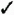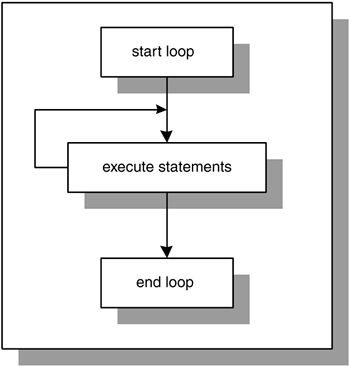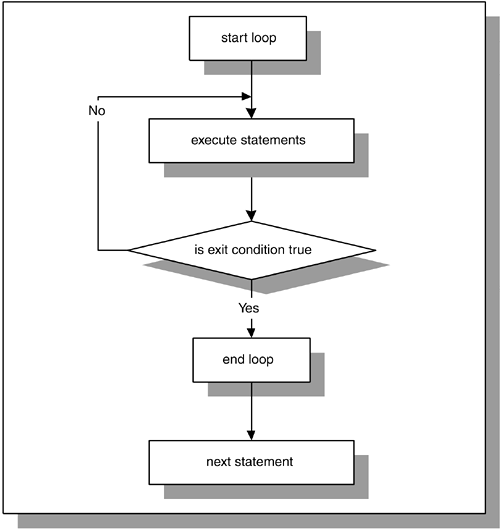# Lab 8.1 Simple Loops

## Lab Objectives

After this Lab, you will be able to:Use Simple Loops with EXIT ConditionsUse Simple Loops with EXIT WHEN Conditions

A simple loop, as you can see from its name , is the most basic kind of loop and has the following structure:

` `
` LOOP    STATEMENT 1;    STATEMENT 2;    ...    STATEMENT N; END LOOP; `

The reserved word LOOP marks the beginning of the simple loop. Statements 1 through N are a sequence of statements that is executed repeatedly. These statements consist of one or more of the standard programming structures. END LOOP is a reserved phrase that indicates the end of the loop construct.

The flow of logic from this structure is illustrated in Figure 8.1.

##### Figure 8.1. Simple LoopEvery time the loop is iterated, a sequence of statements is executed, and then control is passed back to the top of the loop. The sequence of statements will be executed an infinite number of times, because there is no statement specifying when the loop must terminate. Hence, a simple loop is called an infinite loop because there is no means to exit the loop. A properly constructed loop needs to have an exit condition that determines when the loop is complete. This exit condition has two forms: EXIT and EXIT WHEN.

#### EXIT

The EXIT statement causes a loop to terminate when the EXIT condition evaluates to TRUE. The EXIT condition is evaluated with the help of an IF statement. When the EXIT condition is evaluated to TRUE, control is passed to the first executable statement after the END LOOP statement. This is indicated by the following:

` `
` LOOP    STATEMENT 1;    STATEMENT 2;    IF  CONDITION  THEN       EXIT;    END IF; END LOOP; STATEMENT 3; `

In this example, you can see that after the EXIT condition evaluates to TRUE, control is passed to STATEMENT 3, which is the first executable statement after the END LOOP statement.The EXIT statement is valid only when placed inside of a loop. When placed outside of a loop, it will cause a syntax error. To avoid this error, use the RETURN statement to terminate a PL/SQL block before its normal end is reached as follows : ` ` ` BEGIN DBMS_OUTPUT.PUT_LINE ('Line 1'); RETURN; DBMS_OUTPUT.PUT_LINE ('Line 2'); END; ` This example produces the output: ` ` ` Line 1 PL/SQL procedure successfully completed. ` Because the RETURN statement terminates the PL/SQL block, the second DBMS_OUTPUT.PUT_LINE statement is never executed.

#### EXIT WHEN

The EXIT WHEN statement causes a loop to terminate only if the EXIT WHEN condition evaluates to TRUE. Control is then passed to the first executable statement after the END LOOP statement. The structure of a loop using an EXIT WHEN clause is as follows:

` `
` LOOP    STATEMENT 1;    STATEMENT 2;    EXIT WHEN  CONDITION  ; END LOOP; STATEMENT 3; `

This flow of logic from the EXIT and EXIT WHEN statements is illustrated in Figure 8.2.

##### Figure 8.2. Simple Loop with the EXIT ConditionFigure 8.2 shows that during each iteration, the loop executes a sequence of statements. Control is then passed to the EXIT condition of the loop. If the EXIT condition evaluates to FALSE, control is passed to the top of the loop. The sequence of statements will be executed repeatedly until the EXIT condition evaluates to TRUE. When the EXIT condition evaluates to TRUE, the loop is terminated , and control is passed to the next executable statement following the loop.

Figure 8.2 also shows that the EXIT condition is included in the body of the loop. Therefore, the decision about loop termination is made inside the body of the loop, and the body of the loop, or a part of it, will always be executed at least once. However, the number of iterations of the loop depends on the evaluation of the EXIT condition and is not known until the loop completes.

As mentioned earlier, Figure 8.2 illustrates that the flow of logic for the structure of EXIT and EXIT WHEN statements is the same even though two different forms of EXIT condition are used. In other words,

` `
` IF  CONDITION  THEN    EXIT; END IF; `

is equivalent to

` `
` EXIT WHEN  CONDITION  ; `It is important to note that when the EXIT statement is used without an EXIT condition, the simple loop will execute only once. Consider the following example. ` ` ` DECLARE v_counter NUMBER := 0; BEGIN LOOP DBMS_OUTPUT.PUT_LINE ('v_counter = 'v_counter); EXIT; END LOOP; END; ` This example produces the following output: ` ` ` v_counter = 0 PL/SQL procedure successfully completed. ` Because the EXIT statement is used without an EXIT condition, the loop is terminated as soon as the EXIT statement is executed.Oracle PL[s]SQL by Example
ISBN: 3642256902
EAN: N/A
Year: 2003
Pages: 289